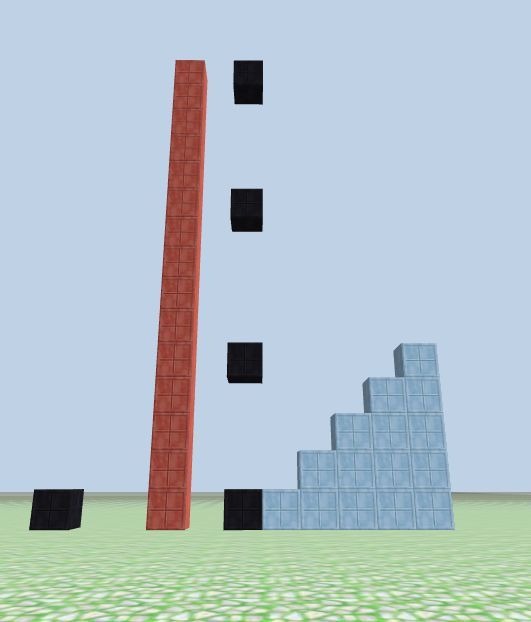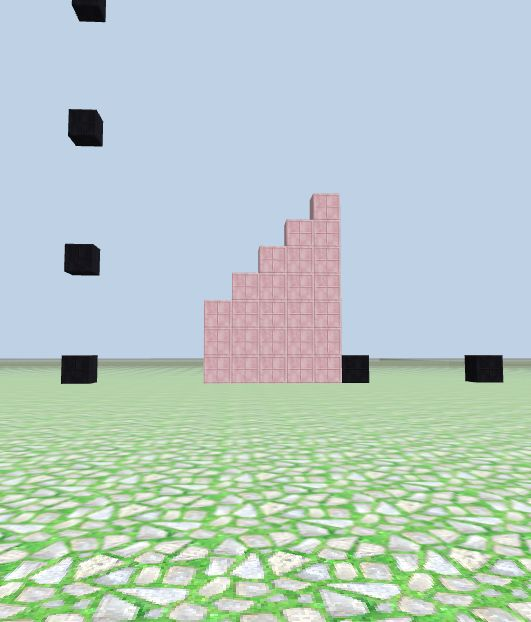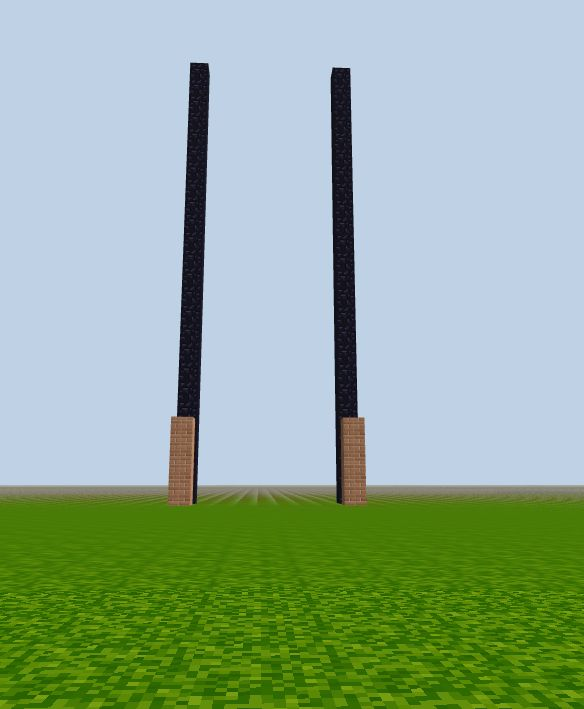# While loop in CodeCraft

### Function `addTo(n)`

Build n columns with heights from 1 to n, then build a tall column that has height the sum of all the numbers, (1 + 2 + ... + n).

``````function addTo(n) {
let sum = 0;
let i = 1;
while (i <= n) {
column(i, -20, i, 'box_light_blue');
sum += i;
i++;
}
column(-2, -20, sum,'box_red');
}

// call the function with input
``````

See in the picture, the numbers `(1,2,3,4,5)` are indicated by blue columns, and the red tall column is the sum of all numbers `(1+2+...+5 = 15)`.Question: can you rewrite this piece of code with a `for` loop?

### Multiple conditions in a while loop

``````// two conditions
let i = 5, h = 3;
while (i < 10 && h < 50) {
column(i, -20, h, 'box_pink');
i++;
h++;
}
``````

`i` (x location) increases from `5` to `9`;
`h` (the column height) increases from `3` to `7`.

In this case, `i` reaches 10 before `h` can reach 50, so `i < 10` limits the loop.### Factorials

We can use a `while` loop to calculate factorials. Do you remember factorials? For example, 4! = 4 * 3 * 2 * 1

``````// while loop to calculate factorial of n
function while_fact() {
let n = prompt('Enter an integer >= 0: ');
let fact = 1, i = 2;

while (i <= n) {
fact *= i;
i++;
}

column(n, -10, n, 'brick');
column(n, -12, fact, 'obsidian');
}

// call the function
while_fact()          // enter n as 4
``````

We can also use `for` loop to calculate factorial:

``````// for loop to calculate factorial of n
function for_fact(n) {
column(-n, -10, n, 'brick');

let fact = 1;
for (let i = 2; i < n+1; i++) {
fact *= i
}
column(-n, -12, fact, 'obsidian');
}

// call the function
for_fact(4)
``````

Run and input the same number for both applications. In this image, you can see that they give the same results: the brick column is n blocks high, and the black column is n! blocks high.